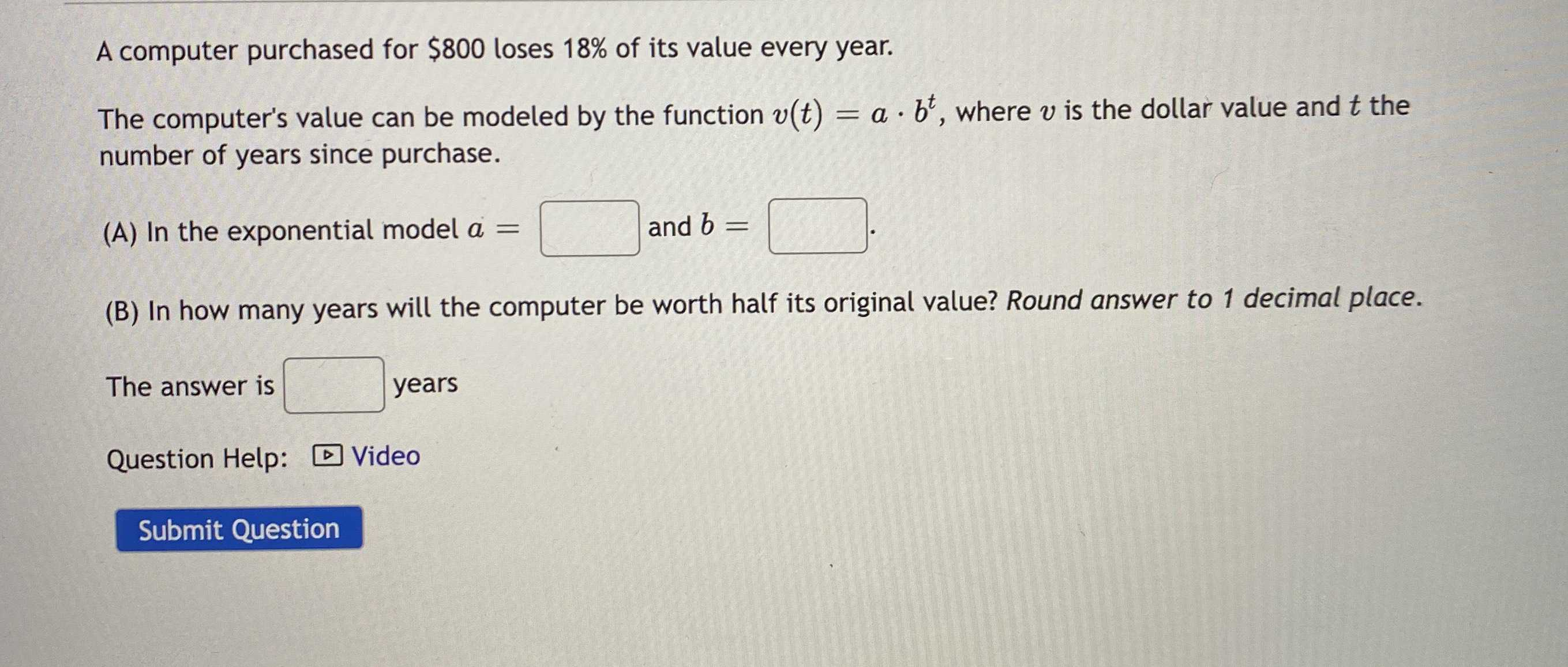### ¿Todavía tienes preguntas de matemáticas?

Pregunte a nuestros tutores expertos
Algebra
PreguntaA computer purchased for $$\ 800$$ loses $$18 \%$$ of its value every year. The computer's value can be modeled by the function $$v ( t ) = a \cdot b ^ { t }$$ , where $$v$$ is the dollar value and $$t$$ the number of years since purchase.

(A) In the exponential model $$a =$$ and $$b =$$

(B) In how many years will the computer be worth half its original value? Round answer to $$1$$ decimal place. The answer is _____years